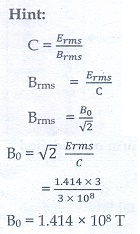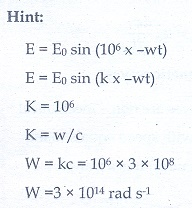Home | | Physics 12th Std | Electromagnetic Waves: Multiple choice questions

# Electromagnetic Waves: Multiple choice questions

Physics : Electromagnetic Waves: Multiple choice questions with answers / choose the correct answer with answers, Physics Book back 1 mark questions and answers with solution

Multiple choice questions

1.The dimension of 1/┬Ą0╬Ą0 is

(a) [L T-1  ]

(b) [L2TŌłÆ2]

(c) [LŌłÆ1 T]

(d) [LŌłÆ2 T2]2. If the amplitude of the magnetic field is 3 ├Ś 10ŌłÆ6 T, then amplitude of the electric field for a electromagnetic waves is

(a) 100 V mŌłÆ1

(b) 300 V mŌłÆ1

(c) 600 V mŌłÆ1

(d) 900 V mŌłÆ13. Which of the following electromagnetic radiation is used for viewing objects through fog

(a) microwave

(b) gamma rays

(c) X- rays

(d) infrared

4. Which of the following are false for electromagnetic waves

a.     transverse

b.     mechanical waves

c.      longitudinal

b.     produced by accelerating charges

5. Consider an oscillator which has a charged particle and oscillates about its mean position with a frequency of 300 MHz. The wavelength of electromagnetic waves produced by this oscillator is

(a) 1 m

(b) 10 m

(c) 100 m

(d) 1000 m6. The electric and the magnetic field, associated with an electromagnetic wave, propagating along X axis can be represented byAns: (b)

7. In an electromagnetic wave in free space the rms value of the electric field is 3 V mŌłÆ1. The peak value of the magnetic field is

(a) 1.414 ├Ś 10ŌłÆ8 T

(b) 1.0 ├Ś 10ŌłÆ8  T

(c) 2.828 ├Ś 10ŌłÆ8 T

(d) 2.0 ├Ś 10ŌłÆ8 T8. During the propagation of electromagnetic waves in a medium:

a.     electric energy density is double of the magnetic energy density

b.     electric energy density is half of the magnetic energy density

c.      electric energy density is equal to the magnetic energy density

d.     both electric and magnetic energy densities are zero

9. If the magnetic monopole exists, then which of the MaxwellŌĆÖs equation to be modified?.Ans: (b)

10. A radiation of energy E falls normally on a perfectly reflecting surface. The momentum transferred to the surface is

(a)E/c

(b)2E/c

(c)Ec

(d)E/c211. Which of the following is an electromagnetic wave?

(a) ╬▒ - rays

(b) ╬▓ - rays

(c) ╬│ - rays

(d) all of them

12.Which one of them is used to produce a propagating electromagnetic wave?.

a.     an accelerating charge

b.     a charge moving at constant velocity

c.      a stationary charge

d.     an uncharged particle

13. Let E = Eo sin[106 x -Žēt] be the electric field of plane electromagnetic wave, the value of Žē is

a.     0.3 ├Ś 10ŌłÆ14 rad sŌłÆ1

b.     3 ├Ś 10ŌłÆ14 rad sŌłÆ1

c.      0.3 ├Ś 1014 rad sŌłÆ1

d.    3 ├Ś 1014 rad sŌłÆ114. Which of the following is NOT true for electromagnetic waves?.

a.     it transport energy

b.     it transport momentum

c.      it transport angular momentum

d.    in vacuum, it travels with different speeds which depend on their frequency

15.The electric and magnetic fields of an electromagnetic wave are

a.     in phase and perpendicular to each other

b.     out of phase and not perpendicular to each other

c.      in phase and not perpendicular to each other

d.     out of phase and perpendicular to each other

Tags : Physics , 12th Physics : Electromagnetic Waves
Study Material, Lecturing Notes, Assignment, Reference, Wiki description explanation, brief detail
12th Physics : Electromagnetic Waves : Electromagnetic Waves: Multiple choice questions | Physics